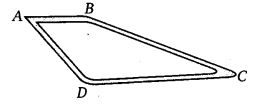# Class 7 Maths NCERT Solutions for Chapter 2 Fractions and Decimals EX – 2.5

## Fractions and Decimals

Question 1.
Which is greater?
(i)
0.5 or 0.05
(ii) 0.7 or 0.5
(iii) 7 or 0.7
(iv) 1.37 or 1.49
(v) 2.03 or 2.30
(vi) 0.8 or 0.88

Solution:
(i) To compare 0.5 and 0.05
Here, the whole number part in each decimal is equal to zero. So, the whole number of parts are equal. Therefore, we will compare the decimal parts. We observe that the extreme left digits in the decimal parts are 5 and 0, respectively such that 5 > 0.
∴ 0.5 > 0.05

(ii) To compare 0.7 and 0.5
Here, the whole number parts are equal, so we compare the decimal parts. In the decimal parts, the extreme left digits are 7 and 5, respectively such that 7 > 5.
∴ 0.7 > 0.5

(iii) To compare 7 and 0.7
The given decimals have distinct whole numbers, so we compare the whole number parts only. These are 7 and 0, respectively such that 7 > 0.
∴ 7 > 0.7

(iv) To compare 1.37 and 1.49
Here, the whole number parts are equal, so we compare the decimal parts. In the decimal parts, the extreme left digits are 3 and 4, respectively such that 3 < 4; i.e., 4 > 3.
∴ 1.49 > 1.37

(v) To compare 2.03 and 2.30
Here, the whole number parts are equal, so we compare the decimal parts. In the decimal parts, the extreme left digits are 0 and 3, respectively such that 0 < 3, i.e., 3 > 0.
∴ 2.30 > 2.03

(vi) To compare 0.8 and 0.88
Here, the whole number parts are equal, so we compare the decimal parts. In decimal part also, digits up to tenths place are equal. So, compare the digits at hundredths place. The digits at hundredths place are 0 and 8 such that 8 > 0.
∴ 0.88 > 0.8

Question 2.
Express as rupees using decimals :
(i) 7 paise
(ii) 7 rupees 7 paise
(iii) 77 rupees 77 paise
(iv) 50 paise
(v) 235 paise

Solution:

Question 3.
(i) Express 5 cm in metre and kilometre
(ii) Express 35 mm in cm, in and km

Solution:

Question 4.
Express in kg :
(i)
200g
(ii) 3470 g
(iii) 4 kg 8 g

Solution:

Question 5.
Write the following decimal numbers in the expanded form :
(i)
20.03
(ii) 2.03
(iii) 200.03
(iv) 2.034

Solution:

Question 6.
Write the place value of 2 in the following decimal numbers :

1. 2.56
2. 21.37
3. 10.25
4. 9.42
5. 63.352

Solution:

1. Place value of 2 in 2.56 is ones.
2. Place value of 2 in 21.37 is tens.
3. Place value of 2 in 10.25 is tenths.
4. Place value of 2 in 9.42 is hundredths.
5. Place value of 2 in 63.352 is thousandths.

Question 7.
Dinesh went from place A to place B and from there to place C. A is 7.5 km from B and B is 12.7 km from C. Ayub went from place A to place D and from there to place C. D is 9.3 km from A and C is 11.8 km from D. Who travelled more and by how much?Solution:

Question 8.
Shyama bought 5 kg 300 g apples and 3 kg 250 g mangoes. Sarala bought 4 kg 800 g oranges and 4 kg 150 g bananas. Who bought more fruits?

Solution:
Shyama bought apples = 5 kg 300 g
Shyama bought mangoes = 3 kg 250 g
Toal fruits bought by Shyama
= 5 kg 300 g + 3 kg 250 g
= 5.300 kg + 3.250 kg
= 8.550 kg

Sarala bought oranges = 4 kg 800 g
Sarala bought banana = 4 kg 150 g
Total fruits bought by Sarala
= 4 kg 800 g + 4 kg 150 g
= 4.800 kg +4.150 kg
= 8.950 kg

Since, 8.950 > 8.550
∴ Sarala bought more fruits.

Question 9.
How much less is 28 km than 42.6 km?

Solution:
Required difference = 42.6 km – 28 km = 14.6 km